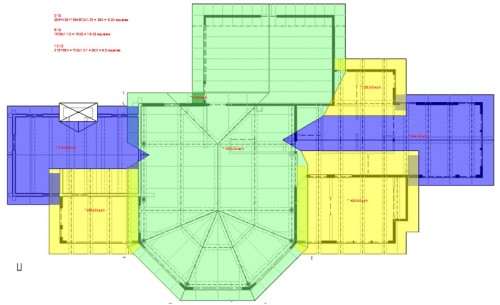# Easy Method to Generate Roof Material Take-offs – Roof Squares

by on May 15, 2017

This follow up article provides a method for generating roof squares of roof material. Builders, contractors and suppliers use “roof squares” as a unit of area for roof materials. All this term really means is that the actual square footage value is divided by 100 and is the unit by which roofing contractors use to price roofing materials.

I use DWF Design Review to help me generate basic square footage values from a roof framing plan and from there I use a roof slope multiplier to account for the slope of the roof and then divide by 100 to arrive at the roof square value.I use various colors to help distinguish between several different roof slopes, for which each slope has a different roof slope multiplier. Once all the areas of a particular slope have been added up, I simply multiply that value by the roof slope multiplier to get the actual square footage of the roof of that particular slope. Then, that value is divided by 100 to get the roof square value. So for example, the areas in green in the graphic above have a roof slope of 6:12 pitch and the area generated by DWF Design Review yields 1,609 sq.ft. And so because we are looking down at the roof, which is sloped, we use a roof slope multiplier of 1.12 to take into account the 6:12 pitch. So 1,609 sq.ft. x 1.12 = 1,802 actual sq. ft. And 1,802 / 100 = 18.02 roof squares.

Here is a table of roof slope multipliers for common roof pitches:

 3 pitch – 1.03 4 pitch – 1.06 5 pitch – 1.085 6 pitch – 1.12 7 pitch – 1.16 8 pitch – 1.21 9 pitch – 1.25 10 pitch – 1.31 11 pitch – 1.36 12 pitch – 1.42